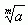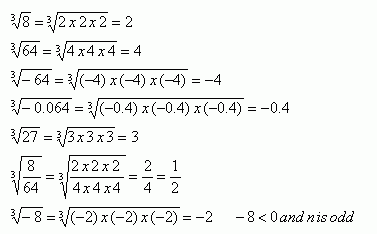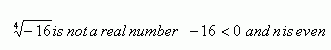Email us to get an instant 20% discount on highly effective K-12 Math & English kwizNET Programs!

#### Online Quiz (WorksheetABCD)

Questions Per Quiz = 2 4 6 8 10

### High School Mathematics2.73 Radicals

 Inm is the index or order Ö is the radical or square root sign a is the radicand Radicals involve the use of the radical sign (√). Sometimes these are also called surds. Square root: A number that must be multiplied times itself to equal a given number. The square root of a is written √a or a1/2.Cube root: A number that must be multiplied times itself three times to equal a given number. The cube root of a is written or 3√a or a1/3.4th root, 5th root or nth root: The number that must be multiplied times itself m times to equal a given value. The mth root of a is written m√a or a1/m. Example, 5√32 = 2 since 25 = 32. Note: When m = 2 an mth root is called a square root. When m = 3 an mth root is called a cube root. When m = 4 an mth root is called fourth root. When m = 5 an mth root is called fifth root. and so on...... If m is even and a is negative, then n√x is non real.A surd or a radical is an expression containing a root with an irrational solution that can not be expressed exactly. For example: √3 = 1.732050808...... For any real number a and any integer m, m>1, the principal nth root of a can be described as follows: If a > 0 thenis a positive real number. If a = 0, thenis zero. If a < 0, and n is odd, thenis a negative real number. If a < 0, and n is even, thenis not a real number. Example: 5Ö3 3 is a positive rational number 5Ö3 is a surd with 5th order. Directions: Choose the correct answer. Also write at least 10 examples of your own for square root, cube root and nth root.
 Q 1: 3Ö10 is a radical expression.TrueFalse Q 2: 3Ö-0.064Answer: Q 3: The index in Ö3 is ____.Answer: Q 4: Ö4/49 = Answer: Q 5: Ö0.09 = ____30.3 Q 6: The order of the surd in 3Ö7 is ______Answer: Q 7: The radicand in 5Ö32 is 5 is ___.Answer: Q 8: The order of the surd in 12Ö3 is ___.Answer: Question 9: This question is available to subscribers only! Question 10: This question is available to subscribers only!

#### Subscription to kwizNET Learning System offers the following benefits:

• Unrestricted access to grade appropriate lessons, quizzes, & printable worksheets
• Instant scoring of online quizzes
• Progress tracking and award certificates to keep your student motivated
• Unlimited practice with auto-generated 'WIZ MATH' quizzes